#Function Repository Resource:

# SelectFirstBy

Apply a function to a list and select the first that satisfies a test

Contributed by: Taliesin Beynon
 ResourceFunction["SelectFirstBy"][test,f,{e1,e2,…}] computes f[ei] until test gives True, or returns \$Failed if none do.

## Examples

### Basic Examples

The first factorial value greater than 1,000:

 In:=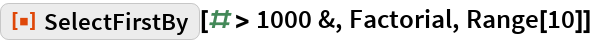Out=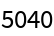If there are no elements satisfying a criterion, SelectFirstBy gives \$Failed:

 In:=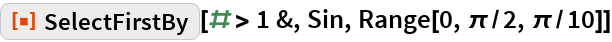Out=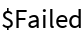### Applications

The first element that satisfies an approximation according to some criteria:

 In:=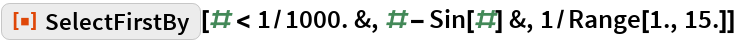Out=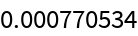The first word consisting of 20 letters:

 In:=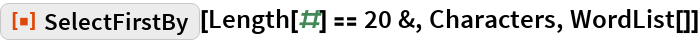Out=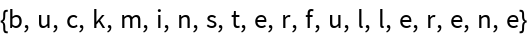The first element with a melting point greater than 1,000 degrees:

 In:=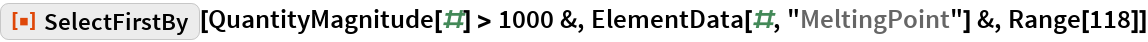Out=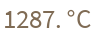### Properties and Relations

SelectFirst uses a slightly different syntax:

 In:=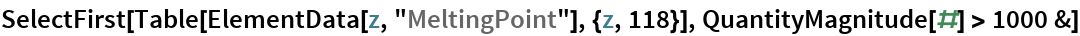Out=## Requirements

Wolfram Language 11.3 (March 2018) or above